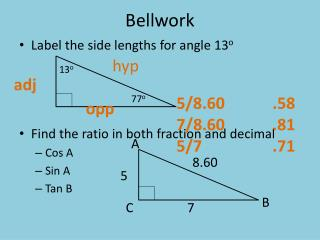DownloadDownload PresentationBellwork

# Bellwork

Download Presentation## Bellwork

- - - - - - - - - - - - - - - - - - - - - - - - - - - E N D - - - - - - - - - - - - - - - - - - - - - - - - - - -
##### Presentation Transcript

1. Bellwork hyp • Label the side lengths for angle 13o • Find the ratio in both fraction and decimal • Cos A • Sin A • Tan B 13o adj 77o 5/8.60 .58 7/8.60 .81 5/7 .71 opp A 8.60 5 B C 7

2. Classify each angle as an angle of elevation or angle of depression. 1. 6 2. 9 angle of depression angle of elevation 3. A plane is flying at an altitude of 14,500 ft. The angle of depression from the plane to a control tower is 15°. What is the horizontal distance from the plane to the tower? Round to the nearest foot. 54,115 ft

3. MATHO Review for test FRIDAY

4. On a white piece of paper • Make a 5 by 5 grid • Label a free box • Label the rest of the boxes 1 - 24

5. # 23 11. 17 m # 12 9.04 in #9 6.30 m #8 1.39 m

6. Solve for < H Solve for < G #22 <G = 37o #15 <H = 53o Solve the triangle Solve the triangle Z 9 4 X #24 <C = 68o #11 AC = 1.95 #2 AB = 4.82 #6 XY = 8.06 #19 <X = 26o #7 <Z = 64o Y

7. San Francisco’s Lombard Street is known as one of “the crookedest streets in the world.” The road’s eight switchbacks were built in the 1920s to make the steep hill passable by cars. If the street has a 16% grade, what angle does the street make with the horizontal line? Round to the nearest degree. #3 9o Nate built a skateboard ramp that covers a horizontal distance of 10 ft. The ramp rises a total of 3.5 ft. What angle does the ramp make with the ground? #14 19o A ranger in a lookout tower spots a fire in the distance. The angle of depression to the fire is 12o, and the lookout tower is 40 m tall. What is the horizontal distance to the fire? #20 188 m

8. Solve the triangle #5 <S = 40o # 21 RT = 27.27 # 13 RS = 42.43

9. An observer at the top of a skyscraper sights a tour bus at an angle of depression of 61o. The skyscraper is 910 ft tall. What is the horizontal distance from the base of the skyscraper to the tour bus? 504.42 ft As part of off season training, the Houston Texans football team must sprint up a ramp with a 28 % grade. To the nearest degree, what angle does t his ramp make with a horizontal line? 16 o When the angle of elevation to the sun is 82o, a monument casts a shadow that is 5.1 ft long. What is the height of the monument to the nearest foot? 36.29 ft

10. 1.31 cm 14.30 cm

11. Bellwork • Solve the triangle • Find XY QN = 13.11 <Q = 41o <N = 49o 10.32 cm